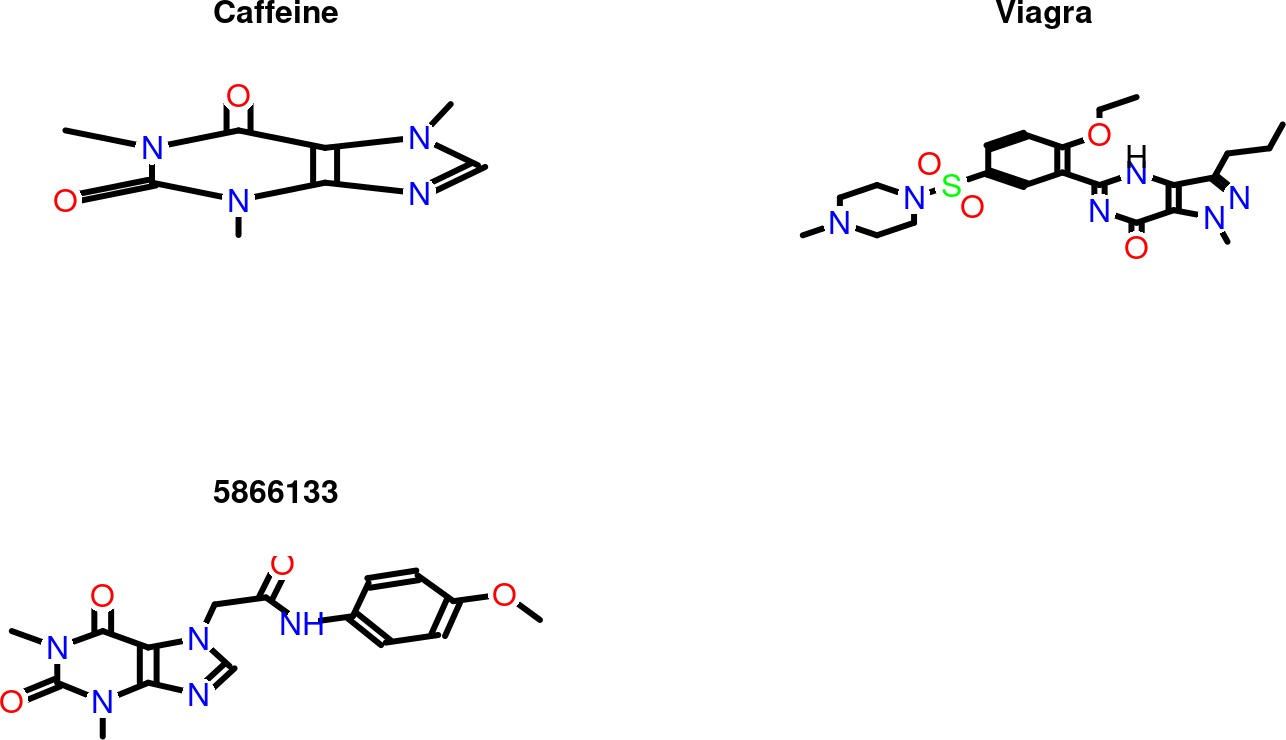Note: the most recent version of this tutorial can be found here and a short overview slide show here.

# 1 Introduction

Maximum common substructure (MCS) algorithms rank among the most sensitive and accurate methods for measuring structural similarities among small molecules. This utility is critical for many research areas in drug discovery and chemical genomics. The MCS problem is a graph-based similarity concept that is defined as the largest substructure (sub-graph) shared among two compounds (Wang et al. 2013; Cao, Jiang, and Girke 2008). It fundamentally differs from the structural descriptor-based strategies like fingerprints or structural keys. Another strength of the MCS approach is the identification of the actual MCS that can be mapped back to the source compounds in order to pinpoint the common and unique features in their structures. This output is often more intuitive to interpret and chemically more meaningful than the purely numeric information returned by descriptor-based approaches. Because the MCS problem is NP-complete, an efficient algorithm is essential to minimize the compute time of its extremely complex search process. The `fmcsR` package implements an efficient backtracking algorithm that introduces a new flexible MCS (FMCS) matching strategy to identify MCSs among compounds containing atom and/or bond mismatches. In contrast to this, other MCS algorithms find only exact MCSs that are perfectly contained in two molecules. The details about the FMCS algorithm are described in the Supplementary Materials Section of the associated publication (Wang et al. 2013). The package provides several utilities to use the FMCS algorithm for pairwise compound comparisons, structure similarity searching and clustering. To maximize performance, the time consuming computational steps of `fmcsR` are implemented in C++. Integration with the `ChemmineR` package provides visualization functionalities of MCSs and consistent structure and substructure data handling routines (Cao et al. 2008; Backman, Cao, and Girke 2011). The following gives an overview of the most important functionalities provided by `fmcsR`.

# 2 Installation

The R software for running `fmcsR` and `ChemmineR` can be downloaded from CRAN (http://cran.at.r-project.org/). The `fmcsR` package can be installed from an open R session using the `BiocManager::install()` command.

``````if (!requireNamespace("BiocManager", quietly=TRUE))
install.packages("BiocManager")
BiocManager::install("fmcsR") ``````

# 3 Quick Overview

To demo the main functionality of the `fmcsR` package, one can load its sample data stored as `SDFset` object. The generic `plot` function can be used to visualize the corresponding structures.

``````library(fmcsR)
data(fmcstest)
plot(fmcstest[1:3], print=FALSE) ``````The `fmcs` function computes the MCS/FMCS shared among two compounds, which can be highlighted in their structure with the `plotMCS` function.

``````test <- fmcs(fmcstest, fmcstest, au=2, bu=1)
plotMCS(test,regenCoords=TRUE) ``````